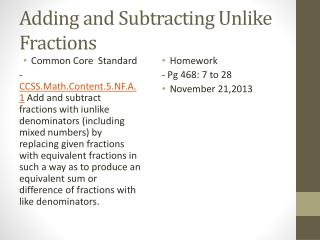DownloadDownload Presentation# Adding and Subtracting Unlike Fractions

Télécharger la présentation## Adding and Subtracting Unlike Fractions

- - - - - - - - - - - - - - - - - - - - - - - - - - - E N D - - - - - - - - - - - - - - - - - - - - - - - - - - -
##### Presentation Transcript

1. Adding and Subtracting Unlike Fractions • Common Core Standard -CCSS.Math.Content.5.NF.A.1 Add and subtract fractions with iunlikedenominators (including mixed numbers) by replacing given fractions with equivalent fractions in such a way as to produce an equivalent sum or difference of fractions with like denominators. • Homework - Pg 468: 7 to 28 • November 21,2013

2. Review • Least Common Denominator/ Least common multiple

3. Finding the Lowest Common Denominator • The lowest common multiple of two numbers is the lowest number in BOTH lists of multiples Multiples of 2 are 2, 4, 6, 8, 10…… Multiples of 3 are 3, 6, 9, 12, ……… What is the lowest common multiple?

4. Finding the Lowest Common Denominator • The lowest common multiple of two numbers is the lowest number they will BOTH divide into 2 divides into 2, 4, 6, 8….. 3 divides into 3, 6, 9…. What is the lowest number 2 and 3 both divide into?

5. You can’t add fractions with different denominators + The Lowest Common Multiple of 2 and 3 is 6 so turn all fractions into sixths Special form of 1

6. Lowest common denominator is 10 so make all fractions tenths

7. Guided Practice

8. Guided Practice

9. Closure • Least Common Denominator/ Multiple • Adding and Subtracting fractions: Write down the steps